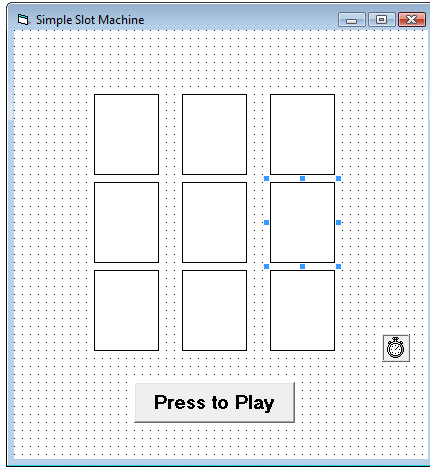# Simple Slot Machine

The slot machines is a game of chance , many different outcomes will appear when the player press the play button. In this program, we draw an array of nine shapes ,VB will automatically labeled the shapes as shape1(0), shape1(1), shape1(2), shape1(3), shape1(4), shape1(5), shape1(6), shape1(7) and shape1(8) respectively. Arrange the shapes into three rows. Write the code so that only three types of shapes appear randomly. Here we want to show only square, oval and rectangle. The appearance can be altered at runtime using the Shape properties. For example, Shape1(o).Shape=0 means it is a rectangle, Shape1(o).Shape=1 is a square and Shape1(o).Shape=2 is an oval shape. The color of the shapes can be customized using the FillColor property of the shape. For example, Shape1(0).Fillcolor=vbRed will give the shape a red color.  The design interface is shown below:Randomness can be achieved by using the Rnd function. We also insert a timer to create the animated effect of a slot machine. The time interval is set to 10 so that the shapes change at a fast rate thus creates the illusion of animation. The program also uses a variable x to control the timer so that it can be stopped when x attain a certain value, otherwise the program will loop forever.

The purpose of this program is just to show how different shapes can appear randomly, therefore many advanced features of a slot machine such as the amount of bet are not programmed here. Those features are available in the professional slot machine.

### The Code

```Private Sub Command1_Click()

'To start the timer
Timer1.Enabled = True
x = 0
End Sub

Private Sub Timer1_Timer()
x = x + 10
Dim a, i As Integer
For i = 0 To 8
' To generate random integers 0,1 and 2
a = Int(Rnd * 3)
Shape1(i).Shape = a
If a = 0 Then
Shape1(i).FillColor = vbRed
ElseIf a = 1 Then
Shape1(i).FillColor = vbGreen
Else
Shape1(i).FillColor = vbBlue
End If
Next i
'To stop the timer
If x > 500 Then
Timer1.Enabled = False
End If

End Sub
```

When you run the program, you will see the following runtime UI:

### The Runtime UI